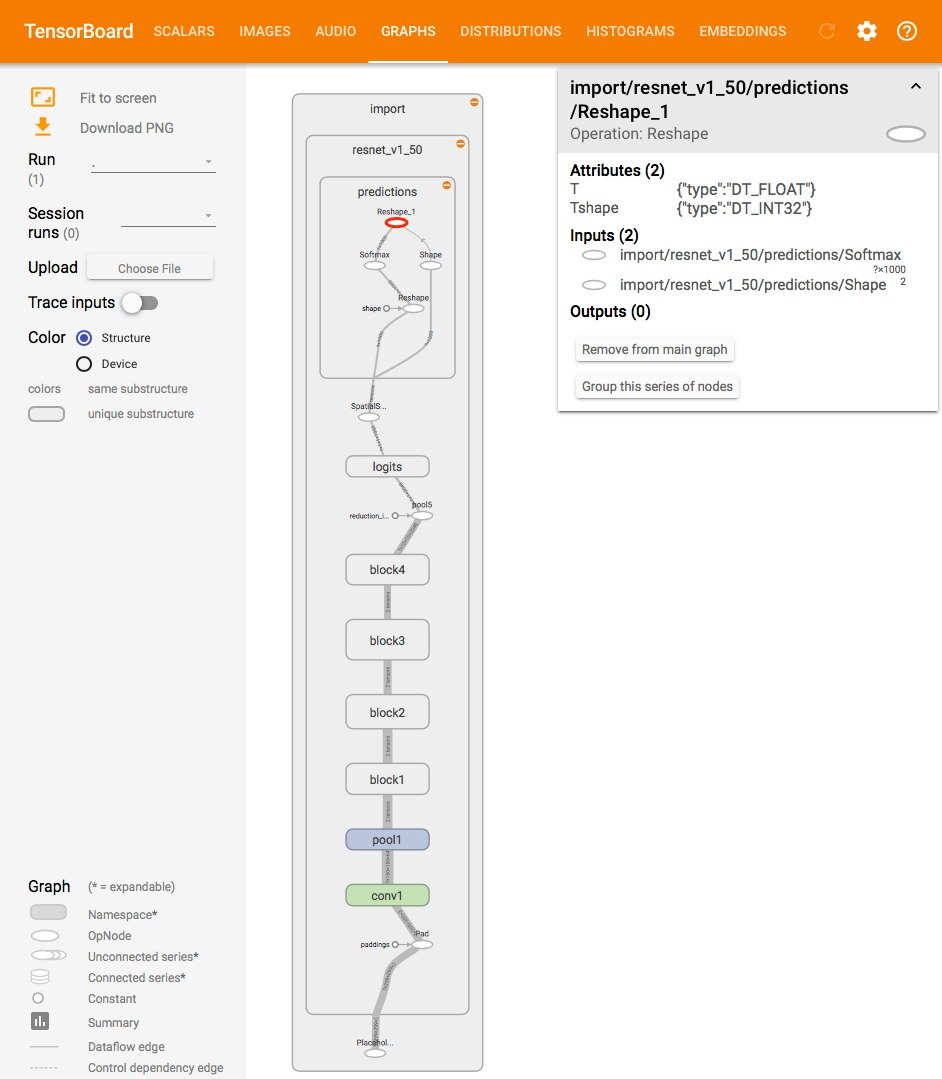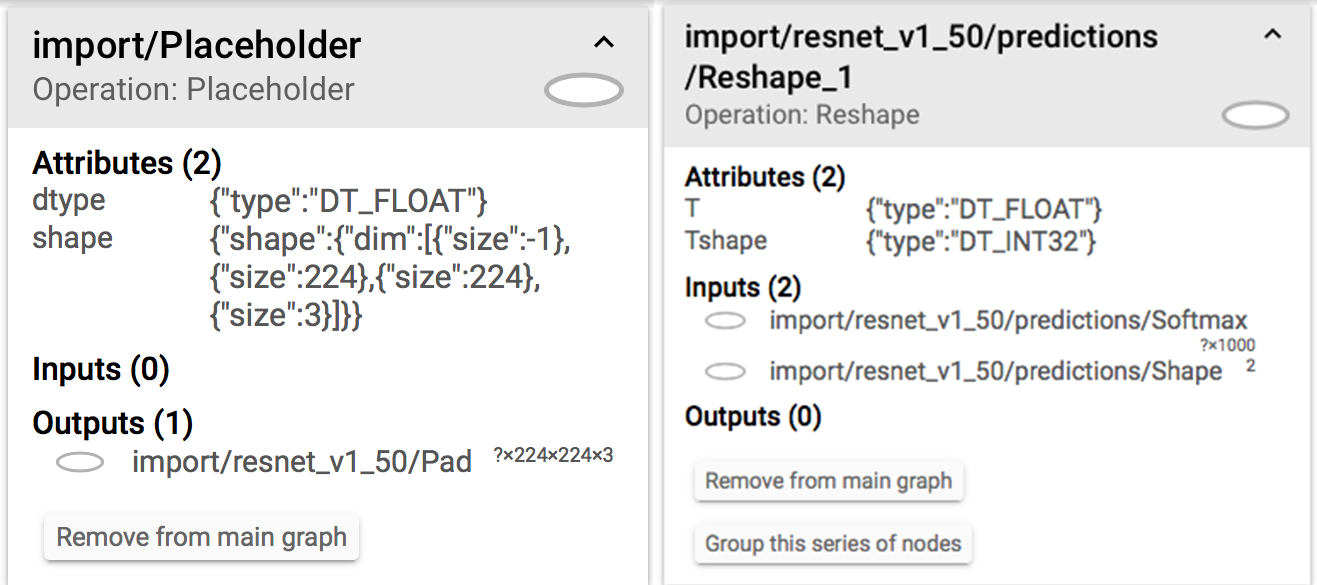## Overview

There are many different ways to deploy a trained neural network model to a mobile or embedded device. Different frameworks support Arm, including TensorFlow, PyTorch, Caffe2, MxNet and CNTK on a variety of platforms, such as Android, iOS and Linux. The deployment process for each is similar but every framework and operating system may use different tools. This walkthrough looks specifically at preparing TensorFlow models for deployment on Android, Linux, and iOS.

Optimization of a trained neural network model with TensorFlow follows these steps:

1. Determine the names of the input and output nodes in the graph and the dimensions of the input data.
2. Generate an optimized 32-bit model using TensorFlow's transform_graph tool.
3. Generate an optimized 8-bit model that is more efficient but less accurate using TensorFlow's transform_graph tool.
4. Benchmark the optimized models on-device and select the one that best meets your deployment needs.

This tutorial goes through each step in turn, using a pretrained ResNet-50 model (resnetv1_50.pb). The process is the same for other models, although input and output node names will differ.

At the end of this tutorial you will be ready to deploy your model on your chosen platform.

## Prerequisites

This tutorial assumes you already have a TensorFlow .pb model file using 32-bit floating point weights. If your model is in a different format (Keras, PyTorch, Caffe, MxNet, CNTK etc) and you want to deploy it using TensorFlow then you'll need to use a tool to convert it to the TensorFlow format first.

There are various projects and resources building up around converting model formats – both MMdnn and Deep learning model converter are useful resources, and the ONNX format has potential to vastly simplify this in the future.

The most important preparation that you can do is to ensure that the size and complexity of your trained model is suitable for the device that you intend to run it on. To ensure this:

• If you are using a pre-trained model for feature extraction or transfer learning, then you should consider using mobile-optimized versions such as MobileNet, TinyYolo and so on.
• If you designed the architecture yourself, then you should consider adapting the architecture for faster execution and smaller size. An example of this is using depth-separable convolutions where possible, as in MobileNet. This provides better performance and accuracy improvements than by post-processing the model file after training.

This tutorial uses TensorFlow's graph_transforms tool, which is built from the TensorFlow source with this command:

`bazel build tensorflow/tools/graph_transforms:transform_graph`

For more details on how to install and build TensorFlow, see the TensorFlow documentation.

## Determine the names of input and output nodes

Skip this step if you are able to determine the names of the input and output nodes from the provider of your model or the training code. However, this step also demonstrates how to visualize the computational graph in a neural network model. This well help you to understand what will be executed at runtime and how the various transform_graph operations affect the structure of the model in practice.

The simplest way to visualize graphs is to use TensorBoard. To install TensorBoard, enter the following on the command line:

`pip install tensorboard`
`tensorboard --logdir=/tmp/tensorboard`

Use the script provided in the TensorFlow source distribution to import model (.pb) files to TensorBoard by entering the following on the command line:

`python tensorflow/python/tools/import_pb_to_tensorboard.py --model_dir resnetv1_50.pb --log_dir /tmp/tensorboard`

Do not let the name of the argument model_dir confuse you. A .pb file is an acceptable target.

Important: if you repeat this command for importing multiple models, empty the /tmp/tensorboard directory after each import to prevent confusion.

In a browser, navigate to http://localhost:6006/ and select the GRAPHS tab to see the model's graph. If it is collapsed under a single node, as shown in this image, use the expand control until you get to the actual operations.In this network we can see that Placeholder is the only input node and Reshape_1 is the output node. To get their full names, select them and look at the details box on the right, as this image shows.Here, the name of the input node is import/Placeholder and the output node is import/resnet_v1_50/predictions/Reshape_1.

The details box also shows that this model has been trained with the standard 224x224x3 input size which is typical for a ResNet architecture. The transform_graph tool assumes the import namespace, so these are simplified to:

• Input name: Placeholder.
• Input dimensions: ?x224x224x3.
• Output name: resnet_v1_50/predictions/Reshape_1.

## Generate an optimized 32-bit model

This step primarily removes unnecessary nodes and ensures that the operations that are used are available in the TensorFlow distributions on mobile devices. One way it does this is by removing training-specific operations in the model's computational graph.

To generate your model, enter the following on the command line:

```bazel-bin/tensorflow/tools/graph_transforms/transform_graph \
--in_graph=resnetv1_50_fp32.pb \
--out_graph=optimized_resnetv1_50_fp32.pb \
--inputs='Placeholder' \
--outputs='resnet_v1_50/predictions/Reshape_1' \
--transforms='strip_unused_nodes(type=float, shape="1,224,224,3")
fold_constants(ignore_errors=true)
fold_batch_norms
fold_old_batch_norms'```

The input and output names will depend on your model. The shape is also included here as part of the strip_unused_nodes command.

If you encounter any problems, the TensorFlow documentation covers this step in more detail.

The transformed model will not differ greatly in size or speed from the training model. The difference is that it is capable of being loaded by the TensorFlow distribution for mobile devices, which may not implement all training operators.

It is a good idea to verify that this inference-ready model runs with the same accuracy as your trained one. How you do this will depend on your training/test workflow and datasets.

You can now distribute this model and deploy it with TensorFlow on both mobile and embedded or low-power Linux devices.

## Generate an optimized 8-bit model

Most trained models use 32-bit floating-point numbers to represent their weights. Research has shown that for many networks you can reduce the precision of these numbers and store them in 8-bit integers, reducing the model size by a factor of 4. This has benefits when distributing and loading models on mobile devices in particular.

In theory, 8-bit integer models can also execute faster than 32-bit floating-point models because there is less data to move and simpler integer arithmetic operations can be used for multiplication and accumulation. However, not all commonly-used layers have 8-bit implementations in TensorFlow 1.4, which means that a quantized model may spend more time converting data between 8-bit and 32-bit formats for different layers than it saves through faster execution.

The optimal balance between speed, size and accuracy will vary by model, application and hardware platform, so it's best to quantize all deployed models and compare them to the unquantized version on the deployment hardware itself. You can quantize a neural network using TensorFlow's graph_transforms tool with the following command:

```bazel-bin/tensorflow/tools/graph_transforms/transform_graph \
--in_graph=resnetv1_50.pb \
--out_graph=optimized_resnetv1_50_int8.pb \
--inputs='Placeholder' \
--outputs='resnet_v1_50/predictions/Reshape_1' \
--transforms='
strip_unused_nodes(type=float, shape="1,224,224,3")
remove_nodes(op=Identity, op=CheckNumerics)
fold_constants(ignore_errors=true)
fold_batch_norms
fold_old_batch_norms
quantize_weights
quantize_nodes
strip_unused_nodes
sort_by_execution_order'```

The quantized network should be significantly smaller than the trained model. In this case, optimized_resnetv1_50_fp32.pb is 97MB whereas optimized_resnetv1_50_int8.pb is 25MB. This can also be important if the model will be distributed as part of a mobile application, quite apart from any inference speed improvements. If size is the primary concern, read the TensorFlow documentation  and try the round_weights transform that reduces the size of the compressed model for deployment without improving speed or affecting accuracy.

Note that it is not currently possible to deploy 8-bit quantized TensorFlow models via CoreML on iOS. It is, however, possible to use the same technique to reduce the compressed model size for distribution using the round_weights transform described in the above link, or to deploy 8-bit models using the TensorFlow C++ interface.

There are several variants of this step, which can include performing extra fine-tuning passes on the model or instrumented runs to determine quantization ranges. To learn more about this and the corresponding deployment workflow with TensorFlow Lite see the TensorFlow Quantization Guide.

## Benchmark the optimized models

It is important to benchmark these models on real hardware. TensorFlow contains optimized 8-bit routines for Arm CPUs but not for x86, so 8-bit models will perform much slower on an x86-based laptop than a mobile Arm device. Benchmarking varies by platform; on Android you can build the TensorFlow benchmark application with this command:

`bazel build -c opt --cxxopt=--std=c++11 --crosstool_top=//external:android/crosstool --cpu=armeabi-v7a --host_crosstool_top=@bazel_tools//tools/cpp:toolchain tensorflow/tools/benchmark:benchmark_model`

With the Android deployment device connected (this example uses a HiKey 960), run:

``adb shell "mkdir -p /data/local/tmp"``
``adb push bazel-bin/tensorflow/tools/benchmark/benchmark_model /data/local/tmp``
``adb push optimized_resnetv1_50_fp32.pb /data/local/tmp``
``adb push optimized_resnetv1_50_int8.pb /data/local/tmp``

The benchmarks are run on a single core (num_threads=1) or four cores (num_threads=4) with these commands:

```adb shell '/data/local/tmp/benchmark_model \
--graph=/data/local/tmp/optimized_resnetv1_50_fp32.pb \
--input_layer="Placeholder" \
--input_layer_shape="1,224,224,3" \
--input_layer_type="float" \
--output_layer="resnet_v1_50/predictions/Reshape_1"'```
```adb shell '/data/local/tmp/benchmark_model \
--graph=/data/local/tmp/optimized_resnetv1_50_int8.pb \
--input_layer="Placeholder" \
--input_layer_shape="1,224,224,3" \
--input_layer_type="float" \
--output_layer="resnet_v1_50/predictions/Reshape_1"'```

Alternatively, deploy the models directly in your application, on iOS, Linux or Android, and use real-world performance measurements to compare the models.

Accuracy should always be evaluated using your own data, as the impact of quantization on accuracy can vary. In terms of compute performance on our HiKey 960 development platform, we see the following:

 Model type Processor Model size 1-batch inference 32-bit floating point Arm Cortex A73 97 MB 1794ms 8-bit integer Arm Cortex A73 25 MB 935ms 32-bit floating point 4x Arm Cortex A73 97 MB 567ms 8-bit integer 4x Arm Cortex A73 25 MB 522ms

### Improving model performance

ResNet-50 on a 224x224x3 image uses around 7 billion operations per inference. It is worth considering whether your application requires a high resolution for fine details in the input, as running ResNet-50 on a 160x160 image would almost halve the number of operations and double the speed. For even faster inference of image processing workloads, investigate performance-optimized models such as the MobileNet family of networks. The MobileNet family allows you to scale computation down by a factor of a thousand, enabling you to scale the model to meet a wide range of FPS targets on existing hardware for a modest accuracy penalty.

## Next steps

The exact deployment method depends on your platform. Use the links in the table to access resources for deploying on your platform:

 Platform Deployment method Android TensorFlow Java or C++ interface iOS CoreML converter (no 8-bit) iOS TensorFlow C++ interface Linux TensorFlow C++ interface